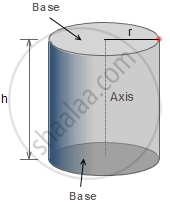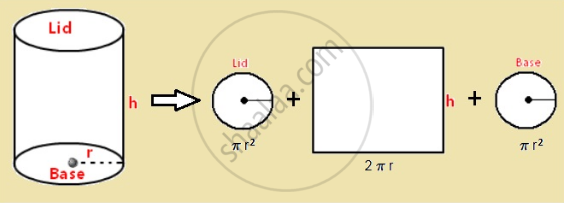# Surface Area and Volume of Cube, Cuboid and Cylinder - Concept of Cylinders

#### notes

A cylinder is a closed solid shape with two parallel bases generally circular in shape connected by two parallel sides. A cylinder may also be defined as a rectangle with circular bases. These circular bases are always parallel and congruent to one another.

A cylinder when unrolled gives a flattened look of a rectangle. Base and sides together form a cylinder. The line joining the two circular bases at the center is the axis of the cylinder. Height (h) as seen in the figure is the perpendicular distance between the two bases. Each base has a radius (r).Curved Surface area:
the length of the cylinder = the circumference of the base = 2πr. The breadth of the rectangle = Height of the cylindrical can = h.

Now, since the rectangular paper was used to wrap the Can firmly, we know that area of rectangle = area of the curved surface of the Cylindrical Can = l × b = 2πr × h

The Curved Surface Area of the Cylinder = 2πrh

Surface area:
We already know that a cylindrical shape is a combination of two circular bases and rectangle like shape that joins the two bases. So, the total surface area of this geometrical figure shall include the area of the two bases and the curved surface area.

Hence, the total surface area =

Area of base 1 +Area of base 2+ Curved surface area
= πr^2 +πr^2 + 2πrh
= 2πr^2 + 2πrh
= 2πr (r+h).
Thus,The Surface Area of a Cylinder = 2πr (r+h)If you would like to contribute notes or other learning material, please submit them using the button below.